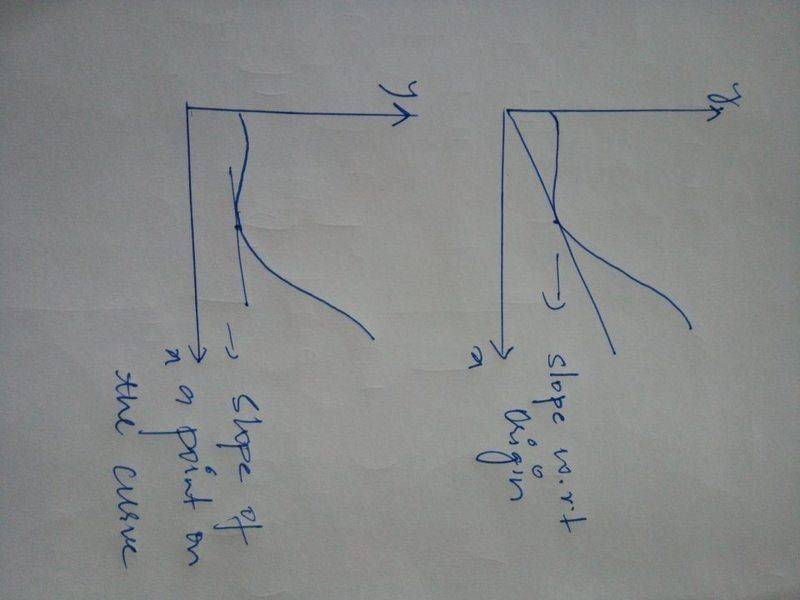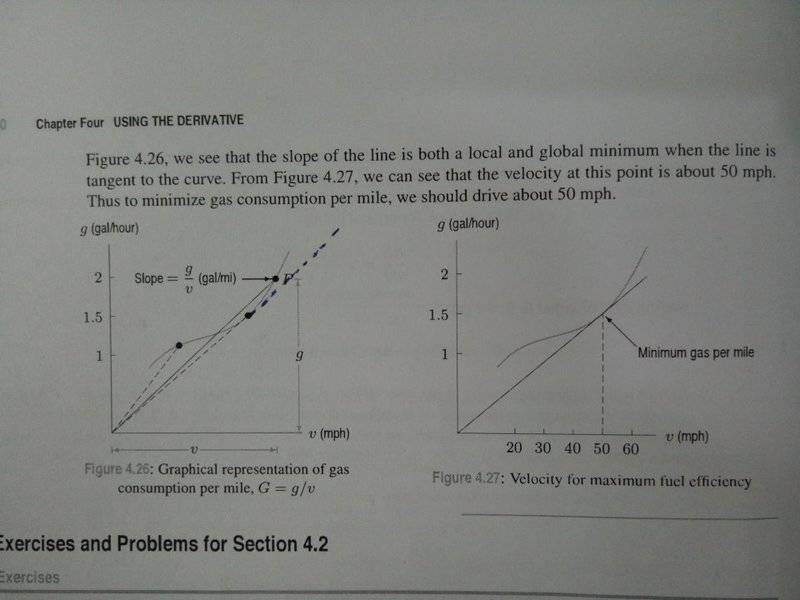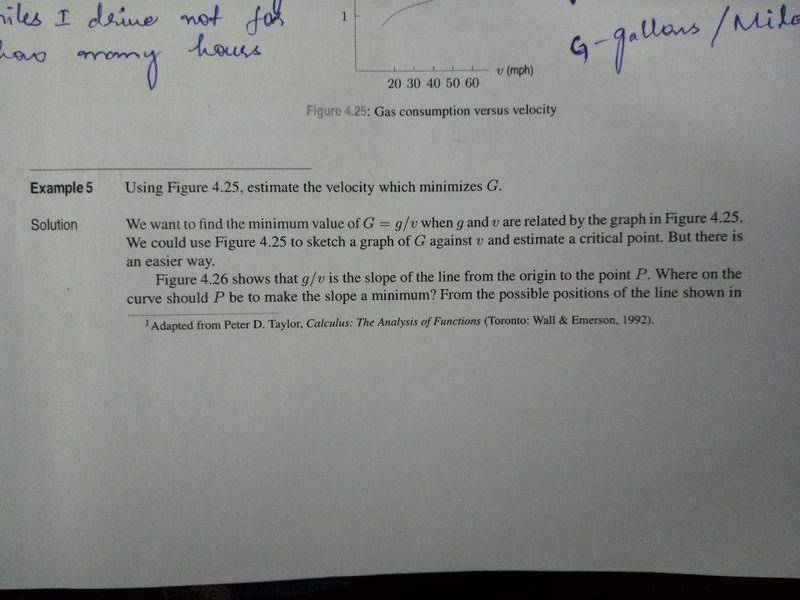# Slope w.r.t Origin: Difference, Usage & Examples

• I
• Mayank Totloor
They're comparing the slopes of the various segments from the origin to the points on the curve. The slope of the tangent line at the left-most point is less than at the other two points shown in this graph, but that's not what they're comparing. They're comparing the slopes of the various segments from the origin to the points on the curve.f

#### Mayank Totloor

What is the difference between slope w.r.t origin and slope at a point on the curve and when are they used ?"Slope with respect to the origin" is not a very useful concept, I don't believe. In the drawing on the right, the curve you drew might as well not be there. All you are doing is measuring the slope of the line segment between the origin and some point. The slope at a point on a curve is very useful, as it gives you an idea of the direction of the curve. If a bug were walking along the curve, the tangent line to the curve would point in the same direction the bug is moving. The slope of the tangent line tells you how steeply the curve is rising or falling (or possibly staying level)."Slope with respect to the origin" is not a very useful concept, I don't believe. In the drawing on the right, the curve you drew might as well not be there. All you are doing is measuring the slope of the line segment between the origin and some point. The slope at a point on a curve is very useful, as it gives you an idea of the direction of the curve. If a bug were walking along the curve, the tangent line to the curve would point in the same direction the bug is moving. The slope of the tangent line tells you how steeply the curve is rising or falling (or possibly staying level).
I just came across an example which used slope w.r.t origin. Can you please walk me through the solution

In the photo of your textbook page there is no mention of "slope with respect to the origin." In Fig 4.26, they are comparing the slopes of several line segments going from the origin to various points on the curve. They note that the point at which the slope is smallest is the point for which the tangent line coincides with a line segment from the origin to that point. Does your book actually use this terminology "slope with respect to the origin"? I've never seen it in any textbook.

I still maintain that it is not a very useful concept. The important concept is the slope of the tangent line to a curve at a point on the curve.

In the photo of your textbook page there is no mention of "slope with respect to the origin." In Fig 4.26, they are comparing the slopes of several line segments going from the origin to various points on the curve. They note that the point at which the slope is smallest is the point for which the tangent line coincides with a line segment from the origin to that point. Does your book actually use this terminology "slope with respect to the origin"? I've never seen it in any textbook.

I still maintain that it is not a very useful concept. The important concept is the slope of the tangent line to a curve at a point on the curve.
No, the text does not use the terminology "slope with respect to the origin", now that I've come across this example I thought that there might exist such a concept.
Don't you think the smallest slope is at point where the first line segment meets the curve in the Fig. 4.26 ? (If we consider the slope at a point on the curve).

Here's the question for the previous solution.BTW, the Fig. 4.25 is same as the Fig.4.26

No, the text does not use the terminology "slope with respect to the origin", now that I've come across this example I thought that there might exist such a concept.
Don't you think the smallest slope is at point where the first line segment meets the curve in the Fig. 4.26 ? (If we consider the slope at a point on the curve).
No, but that's a reasonable question. You have to distinguish between "slope at a point on the curve" and "slope of the segment to a point on the curve." In the solution for Example 5, it says, "Figure 4.26 shows that g/v is the slope of the line from the origin to the point P."
In the image of Fig. 4.26, the slope of the tangent line at the left-most point is less than at the other two points shown in this graph, but that's not what they're comparing.

•Mayank Totloor